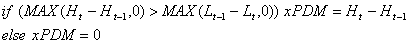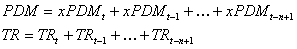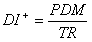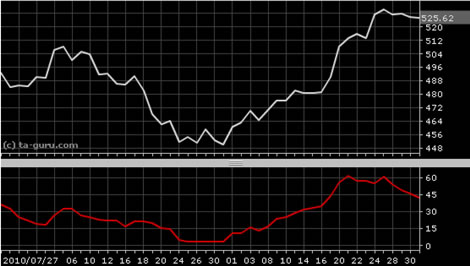Chart Pro online
User name (email):

 Recently used

 Forex

 Indices

# Positive Directional Indicator (DI+)

Positive directional indicator (DI+) is component of average directional index (ADX). If DI+ is sloping upward, it is signal that uptrend is increasing. Indicator was developed by Welles Wilder.

DI+ is calculated by formulas:True range (TR) indicator is used in calculation. For n parameter is usually used value of 14.Positive Directional Indicator (DI+)

Interpretation

Positive directional indicator is usually plotted with negative directional indicator (DI-). For details, see directional movement system (DMS) or average directional index (ADX).

Example